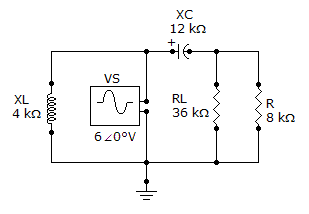Referring to the given circuit, find ZTH if R is 15 kΩ and RL is 38 kΩ.A. 89.82 ∠-51.3° kΩ

B. 19.2 ∠-38.3° kΩ

C. 9.38 ∠-51.3° kΩ

D. 180 ∠-38.3° kΩ

Related Questions on Circuit Theorems In Ac Analysis

The two basic components of a Thevenin equivalent ac circuit are

A. The equivalent voltage source and the equivalent series impedance

B. The equivalent voltage source and the equivalent series resistance

C. The equivalent voltage source and the equivalent parallel impedance

D. The equivalent voltage source and the equivalent parallel resistance